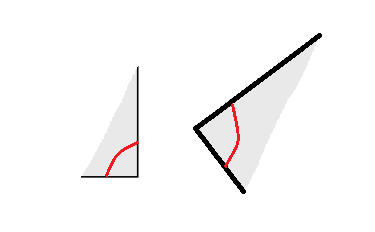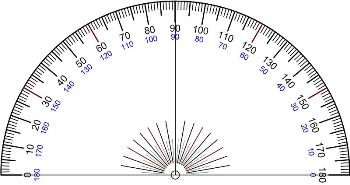What is a Congruent Angle? - Lesson for Kids

Instructor: Mark Boster

Dr. Mark. A. Boster received his doctorate in Educational Leadership from Liberty University and conducted his research in gender roles in award-winning children’s books. He has over 24 years of experience in education at Columbus City Schools holding roles such as curriculum coordinator and classroom teacher.

This lesson is all about congruent angles. You will learn what 'congruent' means, what a congruent angle is and how to tell if one angle is congruent to another angle.

What Are Congruent Angles?

Say you're playing baseball and you hit a ball far into left field. You run to first base then to second, then to third base. What if, when rounding second base on your way to third, you don't turn enough and end up in the field? What if you turn too much and run into the pitcher? Neither of these would be good! So, to avoid running in the wrong direction, you need to turn second base at an angle that's roughly congruent, or the same as, the turn you made at first base.

In math, we say that two angles are congruent angles when they are exactly the same number of degrees--they are open the exact same amount. They can point in completely different directions or be part of completely different-sized shapes--the degrees of the angle just have to be the same. The symbols > and < have congruent angles, and all the 90-degree angles in H, I, and L are congruent.How to Tell if Angles are Congruent

There are a few ways to see if two angles are congruent. The best way is to measure them with a protractor.However, if you don't have a protractor on hand, you might fold a piece of paper so that it fits exactly into one angle and then see if it also fits exactly into the other angle, as well. If it does, the angles are congruent.

To unlock this lesson you must be a Study.com Member.

Register to view this lesson

Are you a student or a teacher?